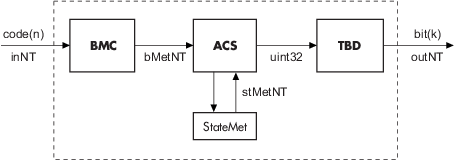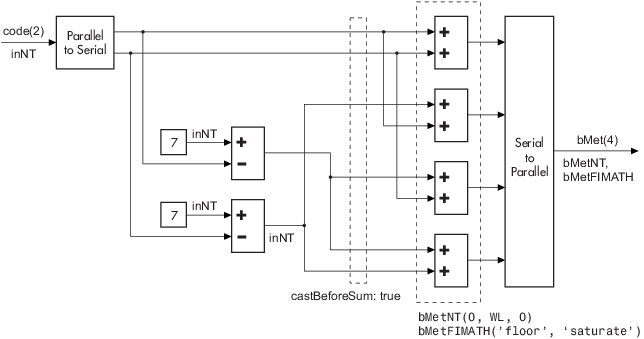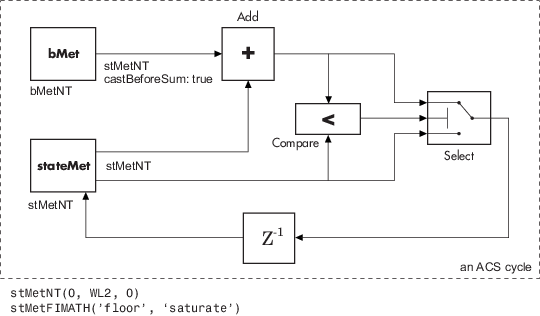Documentation

# Viterbi Decoder

Decode convolutionally encoded data using Viterbi algorithm

## Library

Convolutional sublibrary of Error Detection and Correction## Description

The Viterbi Decoder block decodes input symbols to produce binary output symbols. This block can process several symbols at a time for faster performance.

This block can output sequences that vary in length during simulation. For more information about sequences that vary in length, or variable-size signals, see Variable-Size Signal Basics (Simulink).

### Input and Output Sizes

If the convolutional code uses an alphabet of 2n possible symbols, this block's input vector length is L*n for some positive integer L. Similarly, if the decoded data uses an alphabet of 2k possible output symbols, this block's output vector length is L*k.

This block accepts a column vector input signal with any positive integer value for L. For variable-sized inputs, the L can vary during simulation. The operation of the block is governed by the operation mode parameter.

For information about the data types each block port supports, see the Supported Data Types table on this page.

### Input Values and Decision Types

The entries of the input vector are either bipolar, binary, or integer data, depending on the Decision type parameter.

Decision type ParameterPossible Entries in Decoder InputInterpretation of ValuesBranch metric calculation

`Unquantized`

Real numbers

Positive real: logical zero

Negative real: logical one

Euclidean distance

`Hard Decision`

0, 1

0: logical zero

1: logical one

Hamming distance

`Soft Decision`

Integers between 0 and 2b-1, where b is the Number of soft decision bits parameter.

0: most confident decision for logical zero

2b-1: most confident decision for logical one

Other values represent less confident decisions.

Hamming distance

To illustrate the soft decision situation more explicitly, the following table lists interpretations of values for 3-bit soft decisions.

Input ValueInterpretation
0 Most confident zero
1 Second most confident zero
2 Third most confident zero
3 Least confident zero
4 Least confident one
5 Third most confident one
6 Second most confident one
7 Most confident one

### Operation Modes for Inputs

The Viterbi decoder block has three possible methods for transitioning between successive input frames. The Operation mode parameter controls which method the block uses:

• In `Continuous` mode, the block saves its internal state metric at the end of each input, for use with the next frame. Each traceback path is treated independently.

• In `Truncated` mode, the block treats each input independently. The traceback path starts at the state with the best metric and always ends in the all-zeros state. This mode is appropriate when the corresponding Convolutional Encoder block has its Operation mode set to ```Truncated (reset every frame)```.

• In `Terminated` mode, the block treats each input independently, and the traceback path always starts and ends in the all-zeros state. This mode is appropriate when the uncoded message signal (that is, the input to the corresponding Convolutional Encoder block) has enough zeros at the end of each input to fill all memory registers of the feed-forward encoder. If the encoder has `k` input streams and constraint length vector `constr` (using the polynomial description), “enough” means `k*max(constr-1)`. For feedback encoders, this mode is appropriate if the corresponding Convolutional Encoder block has Operation mode set to ```Terminate trellis by appending bits```.

### Note

When this block outputs sequences that vary in length during simulation and you set the Operation mode to `Truncated` or `Terminated`, the block's state resets at every input time step.

Use the `Continuous` mode when the input signal contains only one symbol.

### Traceback Depth and Decoding Delay

The Traceback depth parameter, D, influences the decoding delay. The decoding delay is the number of zero symbols that precede the first decoded symbol in the output.

• If you set the Operation mode to `Continuous`, the decoding delay consists of D zero symbols

• If the Operation mode parameter is set to `Truncated` or `Terminated`, there is no output delay and the Traceback depth parameter must be less than or equal to the number of symbols in each input.

As a general estimate, the Traceback depth value is approximately two to three times (k – 1)/(1 – r), where k is the constraint length of the code and r is the code rate . For example:

• A rate `1/2` code has a Traceback depth of 5(k – 1).

• A rate `2/3` code has a Traceback depth of 7.5(k – 1).

• A rate `3/4` code has a Traceback depth of 10(k – 1).

• A rate `5/6` code has a Traceback depth of 15(k – 1).

### Reset Port

The reset port is usable only when the Operation mode parameter is set to `Continuous`. Selecting Enable reset input port gives the block an additional input port, labeled `Rst`. When the `Rst` input is nonzero, the decoder returns to its initial state by configuring its internal memory as follows:

• Sets the all-zeros state metric to zero.

• Sets all other state metrics to the maximum value.

• Sets the traceback memory to zero.

Using a reset port on this block is analogous to setting Operation mode in the Convolutional Encoder block to `Reset on nonzero input via port`.

The reset port supports `double` or `boolean` typed signals.

### Fixed-Point Signal Flow Diagram

There are three main components to the Viterbi decoding algorithm. They are branch metric computation (BMC), add-compare and select (ACS), and traceback decoding (TBD). The following diagram illustrates the signal flow for a k/n rate code.As an example of a BMC diagram, a 1/2 rate, nsdec = 3 signal flow would be as follows.`$\begin{array}{c}WL=nsdec+n-1\\ n=2⇒WL=4\end{array}$`

The ACS component is generally illustrated as shown in the following diagram.Where `WL2` is specified on the mask by the user.

In the flow diagrams above, inNT, bMetNT , stMetNT, and outNT are `numerictype` objects, and bMetFIMATH and stMetFIMATH, are `fimath` objects.

## Puncture Pattern Examples

For some commonly used puncture patterns for specific rates and polynomials, see the last three references.

## Fixed-Point Viterbi Decoding Examples

The following two example models showcase the fixed-point Viterbi decoder block used for both hard- and soft-decision convolutional decoding.

If you are reading this reference page in the MATLAB® Help Browser, click Fixed-point Hard-Decision Viterbi Decoding and Fixed-point Soft-Decision Viterbi Decoding to open the models. These can also be found as `doc_fixpt_vitharddec.mdl` and `doc_fixpt_vitsoftdec.mdl` under `help\toolbox\comm\examples`.The layout of the soft decision model example is also similar to the existing doc example on Soft-Decision Decoding, which can be found at `help\toolbox\comm\examples\doc_softdecision.mdl`

The purpose of this model is to highlight the fixed-point modeling attributes of the Viterbi decoder, using a familiar layout.

### Overview of the Simulations

The two simulations have a similar structure and have most parameters in common. A data source produces a random binary sequence that is convolutionally encoded, BPSK modulated, and passed through an AWGN channel.

The Convolutional encoder is configured as a rate 1/2 encoder. For every 2 bits, the encoder adds another 2 redundant bits. To accommodate this, and add the correct amount of noise, the Eb/No (dB) parameter of the AWGN block is in effect halved by subtracting 10*log10(2).

For the hard-decision case, the BPSK demodulator produces hard decisions, at the receiver, which are passed onto the decoder.

For the soft-decision case, the BPSK demodulator produces soft decisions, at the receiver, using the log-likelihood ratio. These soft outputs are 3-bit quantized and passed onto the decoder.

After the decoding, the simulation compares the received decoded symbols with the original transmitted symbols in order to compute the bit error rate. The simulation ends after processing 100 bit errors or 1e6 bits, whichever comes first.

### Fixed-Point Modeling

Fixed-point modeling enables bit-true simulations which take into account hardware implementation considerations and the dynamic range of the data/parameters. For example, if the target hardware is a DSP microprocessor, some of the possible word lengths are 8, 16, or 32 bits, whereas if the target hardware is an ASIC or FPGA, there may be more flexibility in the word length selection.

To enable fixed-point Viterbi decoding, the block input must be of type ufix1 (unsigned integer of word length 1) for hard decisions. Based on this input (either a 0 or a 1), the internal branch metrics are calculated using an unsigned integer of word length = (number of output bits), as specified by the trellis structure (which equals 2 for the hard-decision example).

For soft decisions, the block input must be of type ufixN (unsigned integer of word length N), where N is the number of soft-decision bits, to enable fixed-point decoding. The block inputs must be integers in the range 0 to 2N-1. The internal branch metrics are calculated using an unsigned integer of word length = (N + number of output bits - 1), as specified by the trellis structure (which equals 4 for the soft-decision example).

The State metric word length is specified by the user and usually must be greater than the branch metric word length already calculated. You can tune this to be the most suitable value (based on hardware and/or data considerations) by reviewing the logged data for the system.

Enable the logging by selecting Analysis > Fixed-Point Tool. In the Fixed-Point Setting GUI, set the Fixed-point instruments mode to ```Minimums, maximums and overflows```, and rerun the simulation. If you see overflows, it implies the data did not fit in the selected container. You could either increase the size of the word length (if your hardware allows it) or try scaling the data prior to processing it. Based on the minimum and maximum values of the data, you are also able to determine whether the selected container is of the appropriate size.

Try running simulations with different values of State metric word length to get an idea of its effect on the algorithm. You should be able to narrow down the parameter to a suitable value that has no adverse effect on the BER results.

### Comparisons with Double-Precision Data

To run the same model with double precision data, Select Analysis > Fixed-Point Tool. In the Fixed-Point Tool GUI, select the Data type override to be `Double`. This selection overrides all data type settings in all the blocks to use double precision. For the Viterbi Decoder block, as Output type was set to `Boolean`, this parameter should also be set to double.

Upon simulating the model, note that the double-precision and fixed-point BER results are the same. They are the same because the fixed-point parameters for the model have been selected to avoid any loss of precision while still being most efficient.

### Comparisons Between Hard and Soft-Decision Decoding

The two models are set up to run from within BERTool to generate a simulation curve that compares the BER performance for hard-decision versus soft-decision decoding.

To generate simulation results for `doc_fixpt_vitharddec.mdl`, do the following:

1. Type `bertool` at the MATLAB command prompt.

2. Go to the Monte Carlo pane.

3. Set the Eb/No range to `2:5`.

4. Set the Simulation model to `doc_fixpt_vitharddec.mdl`. Make sure that the model is on path.

5. Set the BER variable name to `BER`.

6. Set the Number of errors to `100`, and the Number of bits to `1e6`.

7. Press and a plot is generated.To generate simulation results for `doc_fixpt_vitsoftdec.mdl`, just change the Simulation model in step 4 and press .

Notice that, as expected, 3-bit soft-decision decoding is better than hard-decision decoding, roughly to the tune of 1.7 dB, and not 2 dB as commonly cited. The difference in the expected results could be attributed to the imperfect quantization of the soft outputs from the demodulator.

## Parameters

Trellis structure

MATLAB structure that contains the trellis description of the convolutional encoder. Use the same value here and in the corresponding Convolutional Encoder block.

Punctured code

Select this check box to specify a punctured input code. The field, Punctured code, appears.

Puncture vector

Constant puncture pattern vector used at the transmitter (encoder). The puncture vector is a pattern of `1`s and `0`s. The `0`s indicate the punctured bits. When you select Punctured code, the Punctured vector field appears.

Enable erasures input port

When you check this box, the decoder opens an input port labeled `Era`. Through this port, you can specify an erasure vector pattern of `1`s and `0`s, where the `1`s indicate the erased bits.

For these erasures in the incoming data stream, the decoder does not update the branch metric. The widths and the sample times of the erasure and the input data ports must be the same. The erasure input port can be of data type `double` or `Boolean`.

Decision type

Specifies the use of `Unquantized`, `Hard Decision`, or ```Soft Decision``` for the branch metric calculation.

• `Unquantized` decision uses the Euclidean distance to calculate the branch metrics.

• `Soft Decision` and ```Hard Decision``` use the Hamming distance to calculate the branch metrics, where Number of soft decision bits equals `1`.

Number of soft decision bits

The number of soft decision bits to represent each input. This field is active only when Decision type is set to `Soft Decision`.

Error if quantized input values are out of range

Select this check box to throw an error when quantized input values are out of range. This check box is active only when Decision type is set to `Soft Decision` or `Hard Decision`.

Traceback depth

The number of trellis branches to construct each traceback path.

Operation mode

Method for transitioning between successive input frames: `Continuous`, `Terminated`, and `Truncated`.

### Note

When this block outputs sequences that vary in length during simulation and you set the Operation mode to `Truncated` or `Terminated`, the block's state resets at every input time step.

Enable reset input port

When you check this box, the decoder opens an input port labeled `Rst`. Providing a nonzero input value to this port causes the block to set its internal memory to the initial state before processing the input data.

Delay reset action to next time step

When you select this option, the Viterbi Decoder block resets after decoding the encoded data. This option is available only when you set Operation mode to `Continuous` and select Enable reset input port. You must enable this option for HDL support.

Output data typeThe output signal's data type can be `double`, `single`, `boolean`, `int8`, `uint8`, `int16`, `uint16`, `int32`, `uint32`, or set to `'Inherit via internal rule'` or ```'Smallest unsigned integer'```.

When set to `'Smallest unsigned integer'`, the output data type is selected based on the settings used in the Hardware Implementation pane of the Configuration Parameters dialog box of the model. If `ASIC/FPGA` is selected in the Hardware Implementation pane, the output data type is `ufix(1)`. For all other selections, it is an unsigned integer with the smallest specified wordlength corresponding to the char value (e.g., `uint8`).

When set to `'Inherit via internal rule'` (the default setting), the block selects double-typed outputs for double inputs, single-typed outputs for single inputs, and behaves similarly to the `'Smallest unsigned integer'` option for all other typed inputs.

## Supported Data Types

PortSupported Data Types

Input

• Double-precision floating point

• Single-precision floating point

• Boolean for `Hard decision` mode

• 8-, 16-, and 32-bit signed integers (for ```Hard decision``` and ```Soft decision``` modes)

• 8-, 16-, and 32-bit unsigned integers (for `Hard decision` and `Soft decision` modes)

• ufix(n), where n represents the Number of soft decision bits

Output

• Double-precision floating point

• Single-precision floating point

• Boolean

• 8-, 16-, and 32-bit signed integers

• 8-, 16-, and 32-bit unsigned integers

• ufix(1) for `ASIC/FPGA` mode

## References

 Clark, G. C. Jr. and J. Bibb Cain., Error-Correction Coding for Digital Communications, New York, Plenum Press, 1981.

 Gitlin, R. D., J. F. Hayes, and S. B. Weinstein, Data Communications Principles, New York, Plenum, 1992.

 Heller, J. A. and I. M. Jacobs, “Viterbi Decoding for Satellite and Space Communication,” IEEE Transactions on Communication Technology, Vol. COM-19, October 1971, pp 835–848.

 Yasuda, Y., et. al., “High-rate punctured convolutional codes for soft decision Viterbi decoding,” IEEE Transactions on Communications, Vol. COM-32, No. 3, pp 315–319, March 1984.

 Haccoun, D., and Begin, G., “High-rate punctured convolutional codes for Viterbi and sequential decoding,” IEEE Transactions on Communications, Vol. 37, No. 11, pp 1113–1125, Nov. 1989.

 Begin, G., et.al., “Further results on high-rate punctured convolutional codes for Viterbi and sequential decoding,” IEEE Transactions on Communications, Vol. 38, No. 11, pp 1922–1928, Nov. 1990.

 Moision, B., “A Truncation Depth Rule of Thumb for Convolutional Codes,” Information Theory and Applications Workshop, pp. 555–557, 2008.

﻿